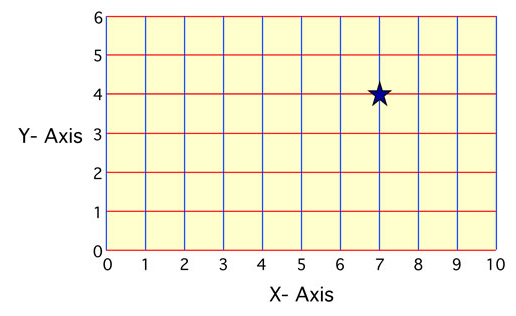# Identifying Grid Location Worksheets

How to Identify a Location on a Grid? Learning the concept of grids is essential when you are trying to grasp the core understanding of graphs. Let us first discuss what actually is a grid? A grid system assists in identifying the location of points traveled from the origin. Let us consider an example:Now, in this scenario, we have to find the distance he took on the x-axis and on the y-axis independently. Let us consider the x-axis first. You can see that it’s above 7, so that means that the star took 7 steps along the x-axis. Now, how many steps did it take towards the y-axis? You can see that the star is in front of 4, so it took 4 steps above.

• ### Basic Lesson

Students are walked through finding location points on an x,y coordinate graph. Identifying the coordinates of items.

• ### Independent Practice 1

Students determine the point location of 10 shapes. The answers can be found below. Determine the location of each shape on the grid and record it next to the shape.

• ### Independent Practice 2

This sheets provides another ten problems for students.

• ### Homework Worksheet

Students are walked through 5 problems and asked to tackle 10 problems on their own.

• ### Skill Quiz

Students write the coordinates of various shapes found a grid.

• ### Homework and Quiz Answer Key

Answers for the homework and quiz.